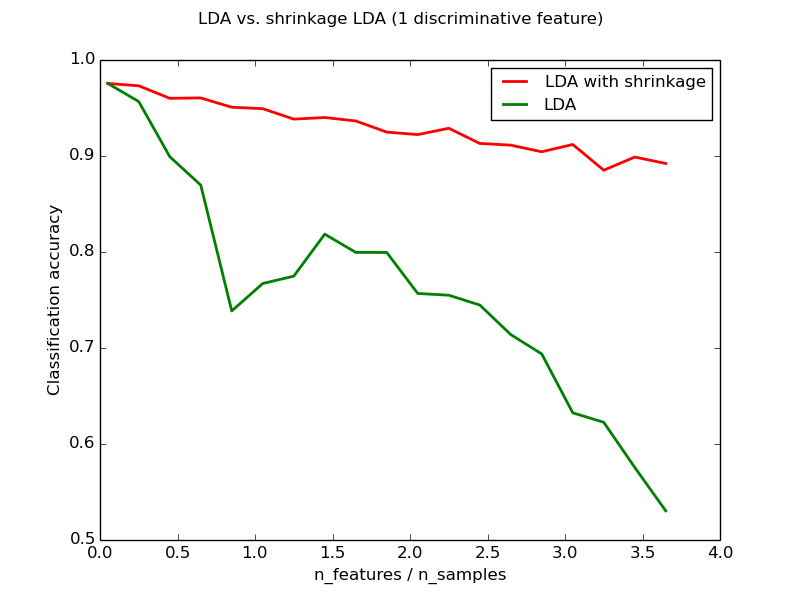This documentation is for scikit-learn version 0.15-gitOther versions

If you use the software, please consider citing scikit-learn.

# Normal and Shrinkage Linear Discriminant Analysis for classification¶

Shows how shrinkage improves classification.Python source code: plot_lda.py

```from __future__ import division

import numpy as np
import matplotlib.pyplot as plt

from sklearn.datasets import make_blobs
from sklearn.lda import LDA

n_train = 20  # samples for training
n_test = 200  # samples for testing
n_averages = 50  # how often to repeat classification
n_features_max = 75  # maximum number of features
step = 4  # step size for the calculation

def generate_data(n_samples, n_features):
"""Generate random blob-ish data with noisy features.

This returns an array of input data with shape `(n_samples, n_features)`
and an array of `n_samples` target labels.

Only one feature contains discriminative information, the other features
contain only noise.
"""
X, y = make_blobs(n_samples=n_samples, n_features=1, centers=[[-2], ])

# add non-discriminative features
if n_features > 1:
X = np.hstack([X, np.random.randn(n_samples, n_features - 1)])
return X, y

acc_clf1, acc_clf2 = [], []
n_features_range = range(1, n_features_max + 1, step)
for n_features in n_features_range:
score_clf1, score_clf2 = 0, 0
for _ in range(n_averages):
X, y = generate_data(n_train, n_features)

clf1 = LDA(solver='lsqr', shrinkage='auto').fit(X, y)
clf2 = LDA(solver='lsqr', shrinkage=None).fit(X, y)

X, y = generate_data(n_test, n_features)
score_clf1 += clf1.score(X, y)
score_clf2 += clf2.score(X, y)

acc_clf1.append(score_clf1 / n_averages)
acc_clf2.append(score_clf2 / n_averages)

features_samples_ratio = np.array(n_features_range) / n_train

plt.plot(features_samples_ratio, acc_clf1, linewidth=2,
label="LDA with shrinkage", color='r')
plt.plot(features_samples_ratio, acc_clf2, linewidth=2,
label="LDA", color='g')

plt.xlabel('n_features / n_samples')
plt.ylabel('Classification accuracy')

plt.legend(loc=1, prop={'size': 12})
plt.suptitle('LDA vs. shrinkage LDA (1 discriminative feature)')
plt.show()
```

Total running time of the example: 5.42 seconds ( 0 minutes 5.42 seconds)